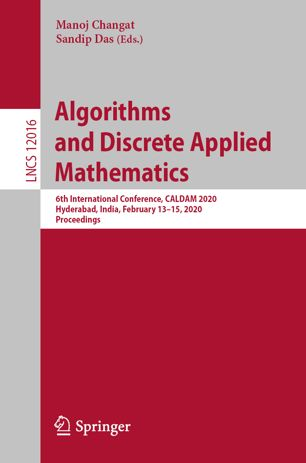• A
• A
• A
• ABC
• ABC
• ABC
• А
• А
• А
• А
• А
Regular version of the site

## Smallest 𝐶_{2𝑙+1}-Critical Graphs of Odd-Girth 2𝑘+1

P. 184-196.
Beaudou L., Foucaud F., Naserasr R.

Given a graph H, a graph G is called H-critical if G does not admit a homomorphism to H, but any proper subgraph of G does. Observe that 𝐾𝑘−1-critical graphs are the classic k-(colour)-critical graphs. This work is a first step towards extending questions of extremal nature from k-critical graphs to H-critical graphs. Besides complete graphs, the next classic case is odd cycles. Thus, given integers 𝑙𝑘 we ask: what is the smallest order 𝜂(𝑘,𝑙) of a 𝐶2𝑙+1-critical graph of odd-girth at least 2𝑘+1? Denoting this value by 𝜂(𝑘,𝑙), we show that 𝜂(𝑘,𝑙)=4𝑘 for 𝑙𝑘≤3𝑙+𝑖−32 (2𝑘=𝑖mod3) and that 𝜂(3,2)=15. The latter is to say that a smallest graph of odd-girth 7 not admitting a homomorphism to the 5-cycle is of order 15 (there are at least 10 such graphs on 15 vertices).

### In bookVol. 12016. Springer, 2020.